#1

## C# EXCEL合併問題

zhi 2020-12-30 10:37:001463 瀏覽

，因為已經卡了二天工時，問資深人員都說沒處理過所以等於沒方向可以找，才上來請教眾大神們，以下為二個XLSX的格式
XLSX File1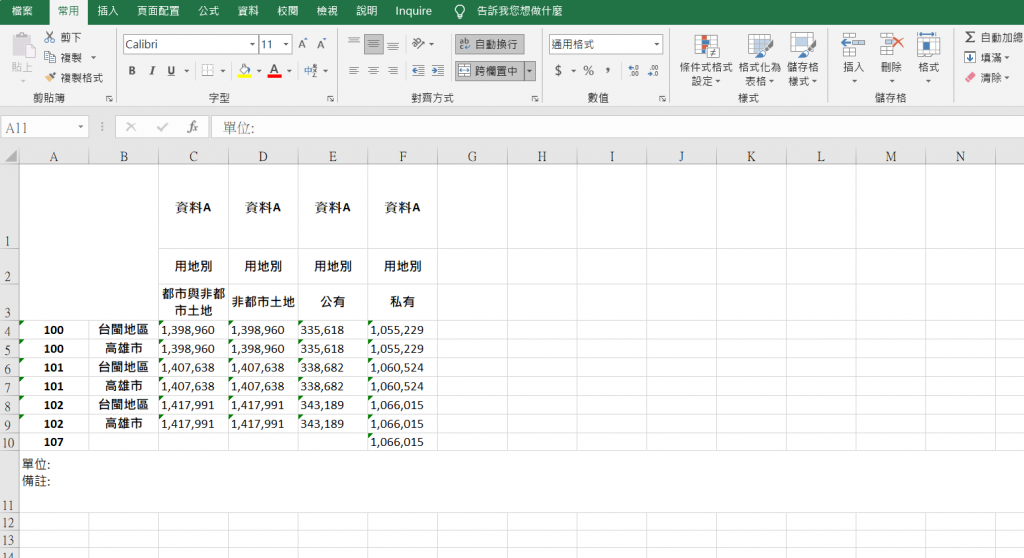XLSX File2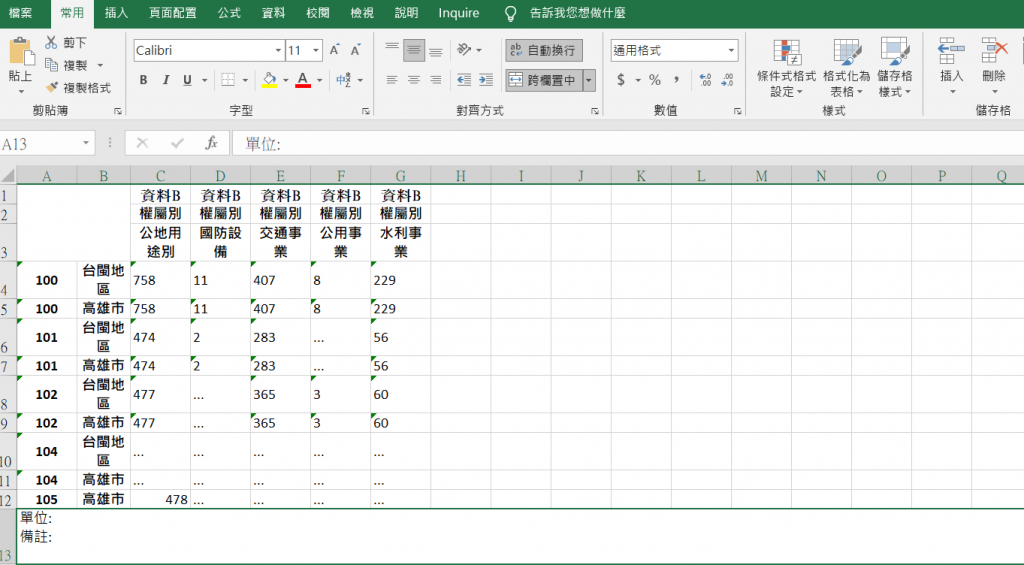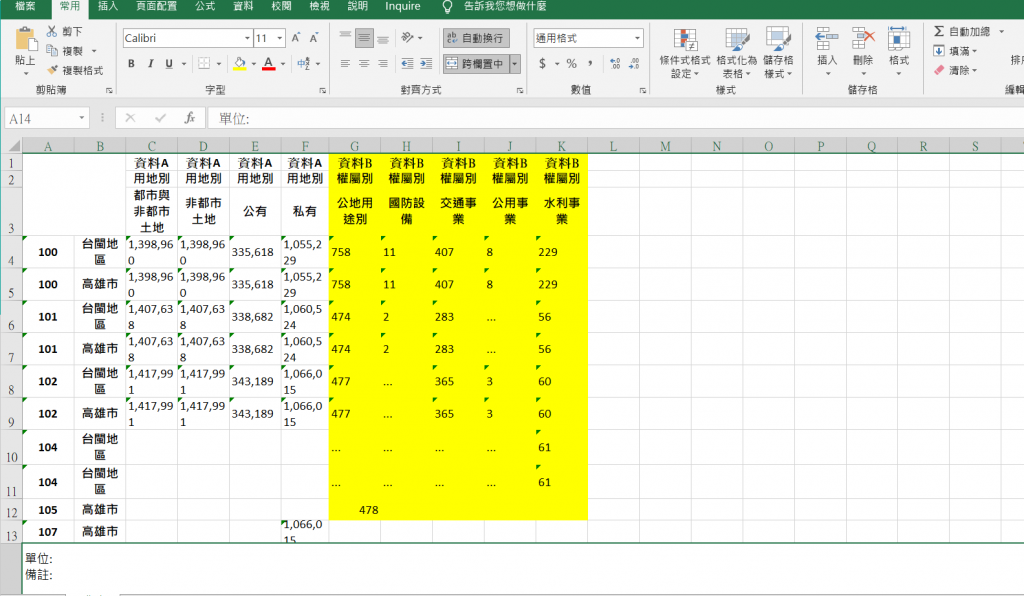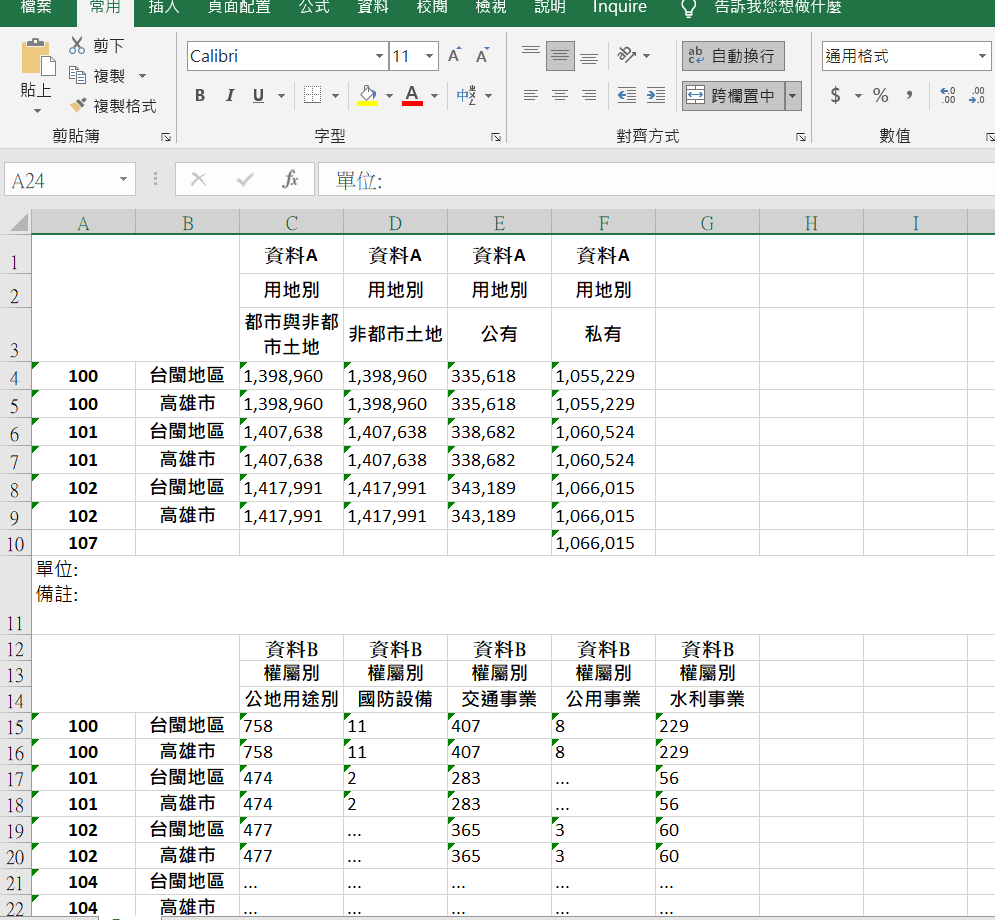zhi iT邦新手 5 級 ‧ 2020-12-30 10:46:08 檢舉

1

### # 程式

``````static void Main(string[] args)
{
//讀取資料的函數
IEnumerable<Hashtable> readData(string fileName, int startRowIndex = 0, int endColIndex = 0)
{
using (var package = new ExcelPackage(new FileInfo(fileName)))
{
var worksheet = package.Workbook.Worksheets.First();

for (var i = startRowIndex; i <= worksheet.Dimension.Rows; i++)
{
var year = worksheet.Cells[i, 1].Text;

//檢查年份，如果年份不對就停止讀取
if (int.TryParse(year, out var r))
{
//不想建立 Class 所以使用 Hashtable
var obj = new Hashtable();
for (var j = 1; j <= endColIndex; j++)
{
}
yield return obj;
}
}
}
}

//讀取資料並轉為字典
startRowIndex: 4, endColIndex: 6)
.ToDictionary(it => new
{
Col1 = it["Col1"].ToString(),
Col2 = it["Col2"].ToString()
});

//讀取資料並轉為字典
startRowIndex: 4, endColIndex: 7)
.ToDictionary(it => new
{
Col1 = it["Col1"].ToString(),
Col2 = it["Col2"].ToString()
});

//產生年份對應表
var result = file1.Concat(file2)
//去除 Key 重複的資料
.Select(it => it.Key).Distinct()
//重新排序
.OrderBy(it => it.Col1).ThenBy(it => it.Col2)
//產生對應表，其餘欄位補空白
.Select(it =>
{
var obj = new Hashtable();
for (var i = 0; i < 9; i++)
return obj;
})
.ToList();

//將兩張表合併
foreach (var item in result)
{
var key = new
{
Col1 = item["Col1"].ToString(),
Col2 = item["Col2"].ToString()
};
//填入表一資料
if (file1.TryGetValue(key, out var o1))
{
for (var i = 1; i <= 4; i++)
item[\$"Col{2 + i}"] = o1[\$"Col{2 + i}"];
}

//填入表二資料
if (file2.TryGetValue(key, out var o2))
{
for (var i = 1; i <= 5; i++)
item[\$"Col{6 + i}"] = o2[\$"Col{2 + i}"];
}
}

//匯出新檔案
using (var package = new ExcelPackage())
{

var rowIndex = 4;

foreach (var item in result)
{
for (var colIndex = 1; colIndex <= 11; colIndex++)
{
worksheet.Cells[rowIndex, colIndex].Value = item[\$"Col{colIndex}"].ToString();
}
rowIndex++;
}

package.SaveAs(new FileInfo("new.xlsx"));
}
}
``````

### # 測試資料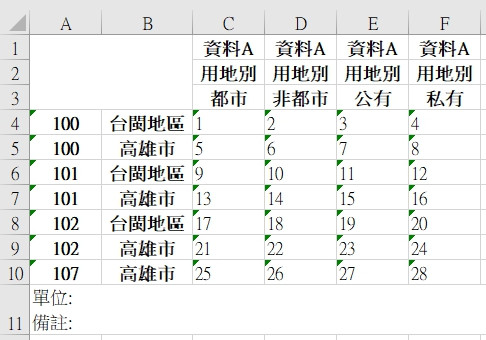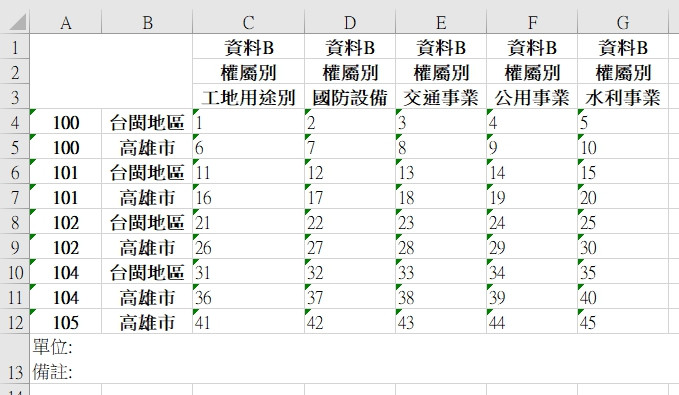### # 結果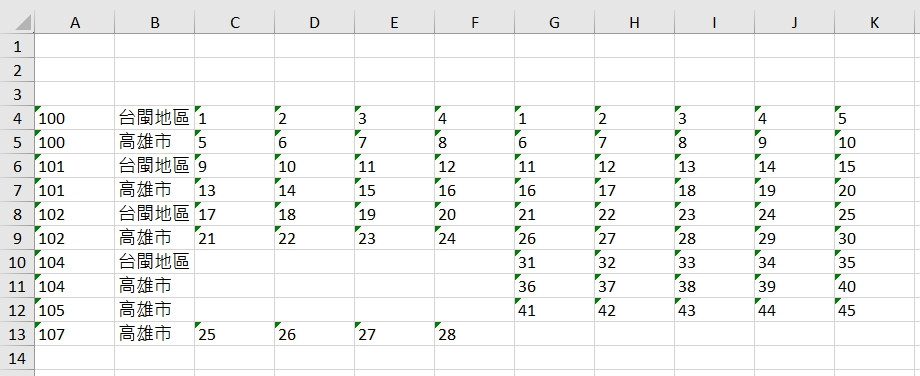zhi iT邦新手 5 級 ‧ 2020-12-30 19:16:03 檢舉

1

1.試著往將xls讀取後，先轉成資料變數。

2.從程式中處理好及整理成你要的格式。

3.再將整理好的資料，重新輸出成一個新的xls。

zhi iT邦新手 5 級 ‧ 2020-12-30 10:48:31 檢舉

zhi iT邦新手 5 級 ‧ 2020-12-30 10:52:54 檢舉

a500197 iT邦新手 5 級 ‧ 2020-12-30 10:59:30 檢舉

zhi iT邦新手 5 級 ‧ 2020-12-30 11:03:43 檢舉

zhi iT邦新手 5 級 ‧ 2020-12-30 11:29:11 檢舉

0

``````using (ExcelPackage epA = new ExcelPackage(new System.IO.FileInfo("a.xlsx")))
{
using (ExcelPackage epB = new ExcelPackage(new System.IO.FileInfo("b.xlsx")))
{
var sheetA = epA.Workbook.Worksheets["sheet1"];
epB.Save();
}
}

``````

zhi iT邦新手 5 級 ‧ 2020-12-30 11:04:04 檢舉

epplus有個優點我更喜歡，用在web server上，讓用戶點擊"匯出"下載excel檔時，無需回寫檔案到硬碟裡，就可以直接on fly下載，省得把我的全SSD網站可憐兮兮的寫入次數給消耗殆盡

``````byte[] respdata = ep.GetAsByteArray();
Response.BinaryWrite(respdata);
Response.Flush();
Response.End();
``````

zhi iT邦新手 5 級 ‧ 2020-12-30 11:28:05 檢舉

``````using (ExcelPackage epA = new ExcelPackage(new System.IO.FileInfo("a.xlsx")))
{
using (ExcelPackage epB = new ExcelPackage(new System.IO.FileInfo("b.xlsx")))
{
foreach(var xs in epA.Workbook.Worksheets)
{
// a_ 的目的只是避免工作表名稱重覆而已，你自己可改慣用命名方法
}
epB.Save("c.xlsx");
//合併後另存成c.xlsx，a跟b.xlsx都不動原稿
}
}

``````

``````var 來源工作表=epA.Workbook.Worksheets;
var 目的工作表=epB.workbook.Worksheets;
int lastRow = 目的工作表.Dimantion.Rows + 3; //空3行
for(int i = 1 ;i<=來源工作表.Dimantion.Rows;i++){
目的工作表.Rows(lastRow+i)=來源工作表.Rows(i);
}
epB.Save("C.xlsx");
``````

zhi iT邦新手 5 級 ‧ 2020-12-30 19:26:09 檢舉

0

zhi iT邦新手 5 級 ‧ 2020-12-30 19:17:17 檢舉